• 累计撰写 164 篇文章
• 累计创建 25 个标签
• 累计收到 208 条评论

### 目 录CONTENT# 实现动态规划2020-09-07 / 0 评论 / 1 点赞 / 278 阅读 / 13,184 字

## 分而治之

### 算法思想

• 分解，将原问题划分为多个子问题。
• 解决，用返回解决子问题的方式的递归算法将子问题解决。
• 组合，组合这些子问题的解决方式，得到原问题的解。

### 实例讲解

• 分解：计算mid并搜索数组较小或较大的一半
• 解决：在较小或较大的一半中搜索值
• 合并：此处我们直接返回了找到的索引值，因此不需要合并

• 因为需要用到递归，所以我们需要两个函数：binarySearchRbinarySearchRecursive，前者用于暴露给开发者调用，后者是分而治之算法。
• 我们先来看看暴露给开发者的binarySearchR 的实现思路：
• 调用排序方法，对待排序数组进行排序
• 声明两个辅助变量lwohigh，分别赋值为0array.length - 1，用于定义分解点，从数组的哪里到哪里执行分而治之方法。
• 调用binarySearchRecursive函数，返回结果。
• 接下来，我们看看分而治之函数binarySearchRecursive的实现思路：
• 它接收四个参数：分割后的数组array、目标值value、数组起始点分解点low、数组末尾分解点high
• 如果low <= high，计算中间值索引mid，取出中间值element。执行下述判断：
• 如果element < value，代表目标值在中间值的右侧，则从mid + 1位置开始分解数组至high位置执行分而治之方法
• 如果element > value，代表目标值在中间值的左侧，则从low位置开始分解数组至mid - 1位置执行分而治之方法
• 否则就是element ===value，目标值找到，返回mid。
• 否则low > high，目标值不在数组中，返回null

// 二分搜索: 递归实现
binarySearchR(): number | null {
this.sort.quickSort();
const low = 0;
const high = this.array.length - 1;
return this.binarySearchRecursive(this.array, this.target, low, high);
}
/**
* 二分搜索递归辅助函数
* @param array 分解后的数组
* @param value 目标值
* @param low 数组起始分解点
* @param high 数组末尾分解点
*/
binarySearchRecursive(array = this.array, value = this.target, low: number, high: number): number | null {
if (low <= high) {
// 计算中间值索引
const mid = Math.floor((low + high) / 2);
// 获取中间值
const element = array[mid];
if (this.compareFn(element, value) === Compare.LESS_THAN) {
// element < value时就从数组的mid+1位置找到high位置，即中间值的右侧
return this.binarySearchRecursive(array, value, mid + 1, high);
} else if (this.compareFn(element, value) === Compare.BIGGER_THAN) {
// element > value时就从数组的low位置找到mid-1位置，即中间值的左侧
return this.binarySearchRecursive(array, value, low, mid - 1);
} else {
// 目标值找到，返回索引
return mid;
}
}
return null;
}


const array = [7, 8, 1, 2, 3, 5, 12, 13, 16, 19];
const searchArithmetic = new SearchArithmetic(array, 7);
const mid = searchArithmetic.binarySearchR();
console.log(mid);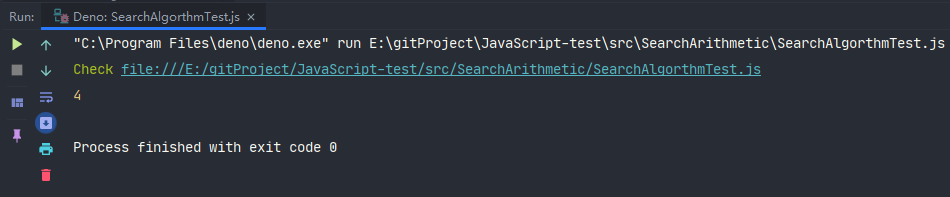## 动态规划

### 算法思想

• 重叠子问题：子问题重复出现
• 最优子结构：一个问题的最优解包含其子问题的最优解
• 无后效性：某阶段的状态一旦确定，则此后过程的演变不再受此前各种状态和决策的影响。

• 将原问题分解成子问题，确定子问题是什么
• 确定状态转移方程，即确定上一个状态和下一个状态之间的关系
• 确定边界条件

### 实例讲解

#### 最少硬币找零问题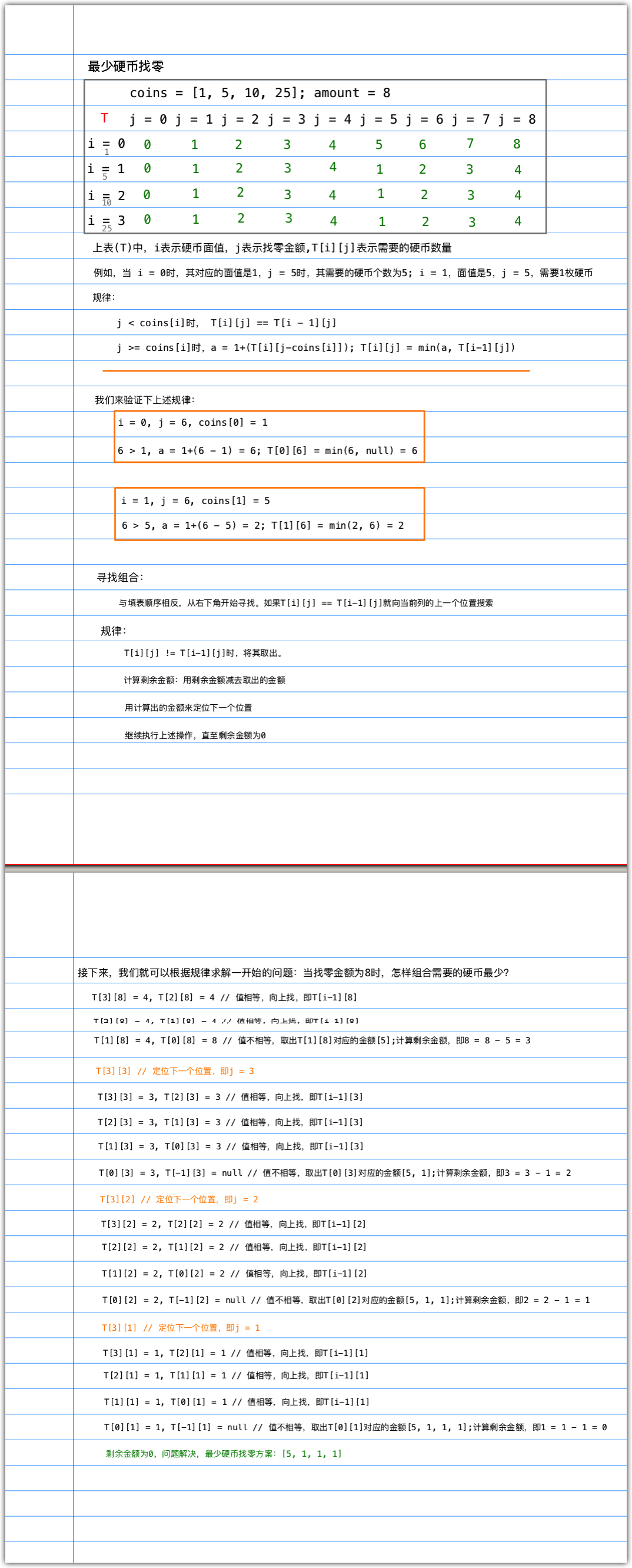• 声明一个函数(minCoinChange)，其接收两个参数：硬币面额coins其类型为数组，找零总金额amount其类型为数字
• 声明一个二维数组cache用于存储已经找到的组合，防止递归计算时遇到已经计算过一遍出组合的金额再次重复计算，从而提升算法的时间复杂度。这一技巧称之为记忆化技巧
• 函数内部声明递归函数（makeChange），其接受一个参数找零金额amount，用于将大问题划分为小问题，最终得到总问题的答案，函数内部实现思路如下。
• 首先，我们要确定递归的终止条件，即amount == false的时候
• 其次，判断当前amount是否已经计算出组合。即cache[amount]true，如果为true则直接返回cache中存储的组合。
• 声明我们接下来我们需要的三个变量：最小组合min、上一层递归计算出来的最小组合newMin、需要再次进行递归的金额newAmount
• 随后，遍历coins数组，递归将大问题划分成小问题，得到最终答案。
• 获取当前遍历到的面值，即coin = coins[i]
• amount - coin就是newAmount的值
• 如果计算出来的newAmount的值大于等于0就递归，即加入递归栈中
• 递归执行完成后，我们依次取出递归栈中的数据，判断其值是否满足拼接条件，如果满足就取出当前递归栈中存储的coin的值，将其与newMin进行拼接，将结果赋值给min
• 遍历结束，将min赋值给cache[amounr]，即找到的组合放进缓存中，将其结果返回，其结果就是当前栈的返回值，即newMin的值。
• 递归函数执行完毕，最终答案已得到，将其返回。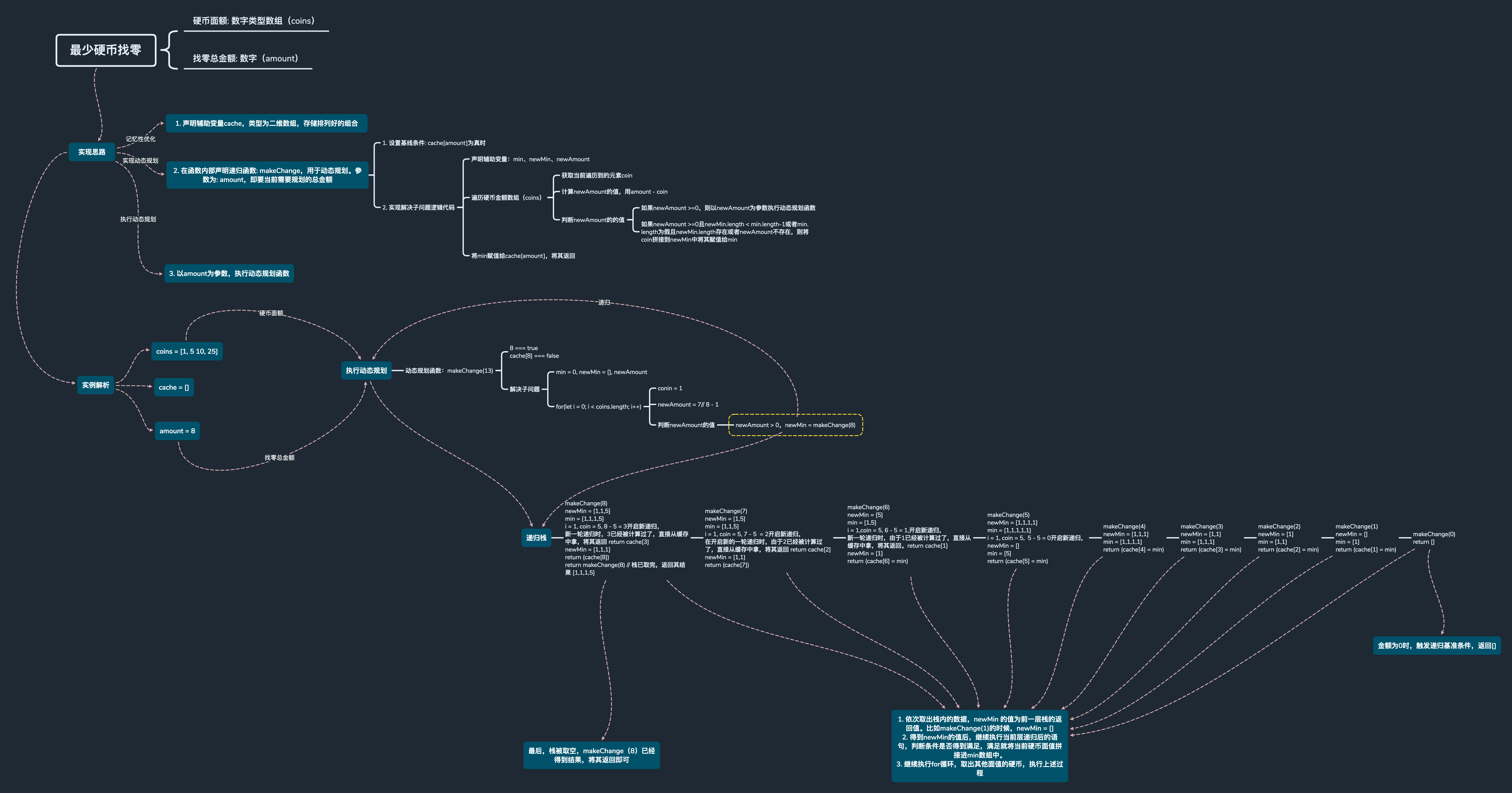##### 实现代码

/**
*
* @param coins 硬币面额
* @param amount 找零总金额
*/
minCoinChange(coins: number[], amount: number): number[] {
// 记忆化技巧，目的是更小且不重复计算值
const cache: number[][] = [];
const makeChange = (amount: number) => {
// 如果amount为false时直接返回空数组
if (!amount) {
return [];
}
// 如果结果已缓存则直接返回结果
if (cache[amount]) {
return cache[amount];
}
let min: number[] = [],
newMin: number[] = [],
newAmount: number;
for (let i = 0; i < coins.length; i++) {
const coin = coins[i];
newAmount = amount - coin;
if (newAmount >= 0) {
// 将newAmount加入递归栈
newMin = makeChange(newAmount);
}
// 递归执行完成，条件如果满足，就将当前硬币
if (newAmount >= 0 && (newMin.length < min.length - 1 || !min.length) && (newMin.length || !newAmount)) {
// 取出当前递归栈中保存的coin值，将其与newMin数组进行拼接，将结果赋值给min
min = [coin].concat(newMin);
}
}
// 将min赋值给cache[amount]，将结果返回，其结果就是当前栈的返回值，即newMin的值
return (cache[amount] = min);
};
return makeChange(amount);
}


##### 编写测试代码

import { DesignSkills } from "./lib/DesignSkills.ts";
const designSkills = new DesignSkills();
const result = designSkills.minCoinChange([1, 5, 10, 25], 8);
console.log(result);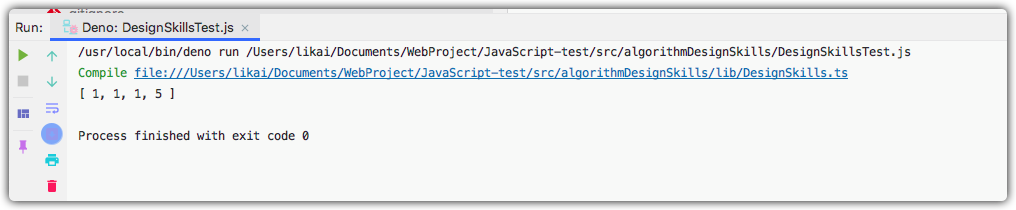#### 背包问题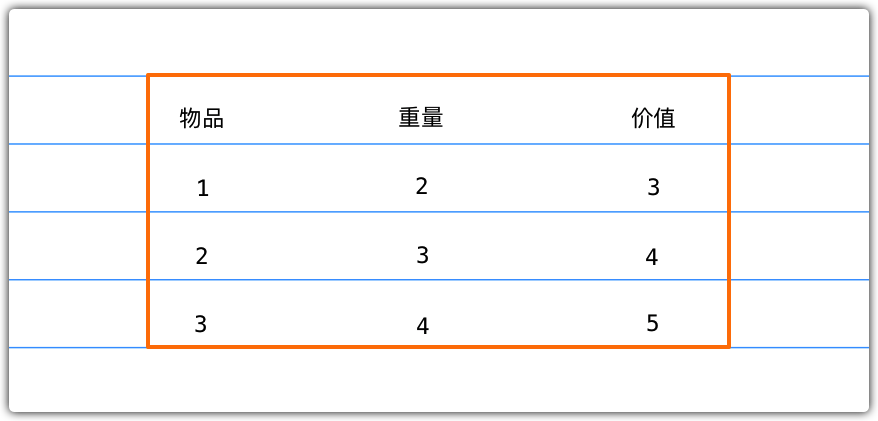• 构造矩阵
• 根据矩阵推出组合

1. 声明并初始化kS二维表格，即矩阵
2. 遍历所有物品(n)，即i <= n
遍历背包容量(capacity)，开始填充背包，即w <= capacity
填充规则如下：
(1). 当i == 0 || w == 0时， 则kS[i][w] = 0
(2). 当物品重量(weights)的i-1位置的元素小于等于w，即weights[i-1] <= w则声明两个辅助变量a和b，a = values[i - 1] + kS[i-1][w-weights[i-1]]b = kS[i-1][w]求出其值后取a和b的最大值作为KS[i][w]的值，即kS[i][w] = max(a,b).
(3). 当重量超出背包能够携带的重量时，则kS[i][w] = kS[i-1][w]

• i = 0,w = 0时，kS = 0
• i = 0, w = 1时，kS = 0
• i = 0, w = 2时，kS = 0
• i = 0, w = 3时，kS = 0
• i = 0, w = 4时，kS = 0
• i = 0, w = 5时，kS = 0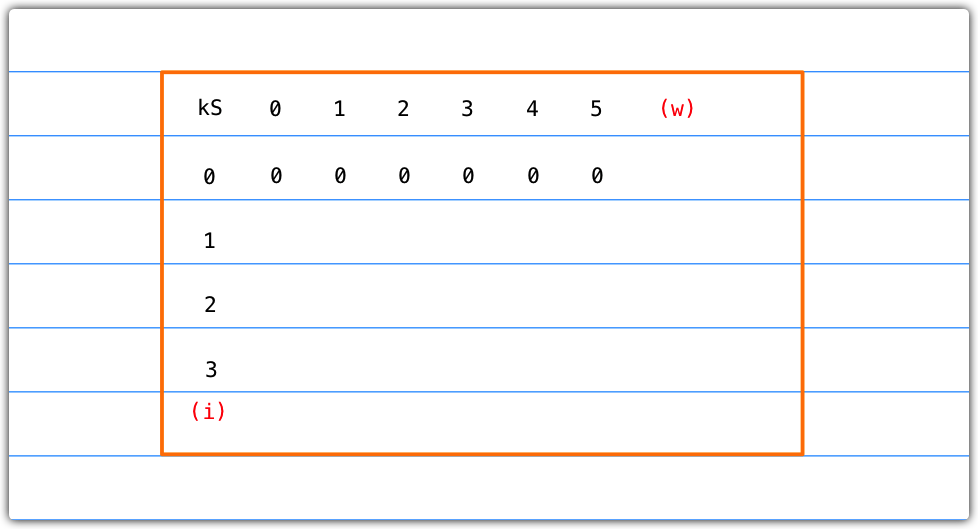• i = 1, w = 0时，kS = 0
• i = 1, w = 1时，weights[1-1] = 2; 2 > w, kS[i][j] = kS[i-1][w] = kS = 0
• i = 1, w = 2时，weights[i-1] = 2, 2 = w, a = values[1-1] + kS[1-1][2 - weights[1-1]] = values + kS[2 - 2] = 3 + 0 = 3;b = kS[1-1][w] = 0; kS[i][w] = max(3,0) = 3
• i = 1, w = 3时，weights[i-1] = 2, 2 < w, a = values[1-1] + kS[1-1][3 - weights[1-1]] = values + kS[3-2] = 3 + 0 = 3; b = kS[1-1][w] = 0; kS[i][w] = max(3,0) = 3
• i = 1, w = 4时，weightd[i-1] = 2, 2 = 2, a = values[1-1] + kS[1-1][4 - weights[1-1]] = 3 + 0 = 3; b = kS[1-1][w] = 0; kS[i][w] = max(3,0) = 3
• i = 1, w = 5时，weights[i-1] = 2, 2 < 2, a = values[1-1] + kS[i-1][5 - weights[1-1]] = 3 + 0 = 3; b = kS[1-1][w] = 0; kS[i][w] = max(3,0) = 3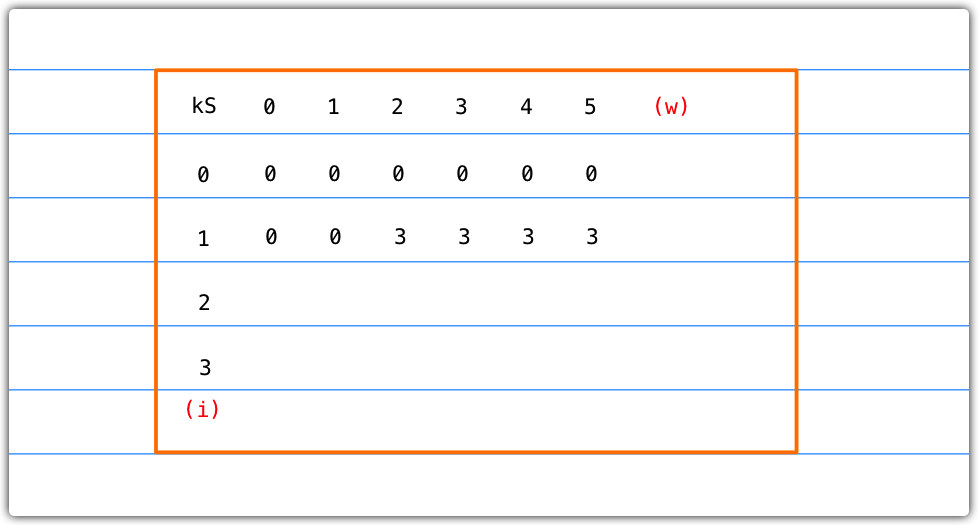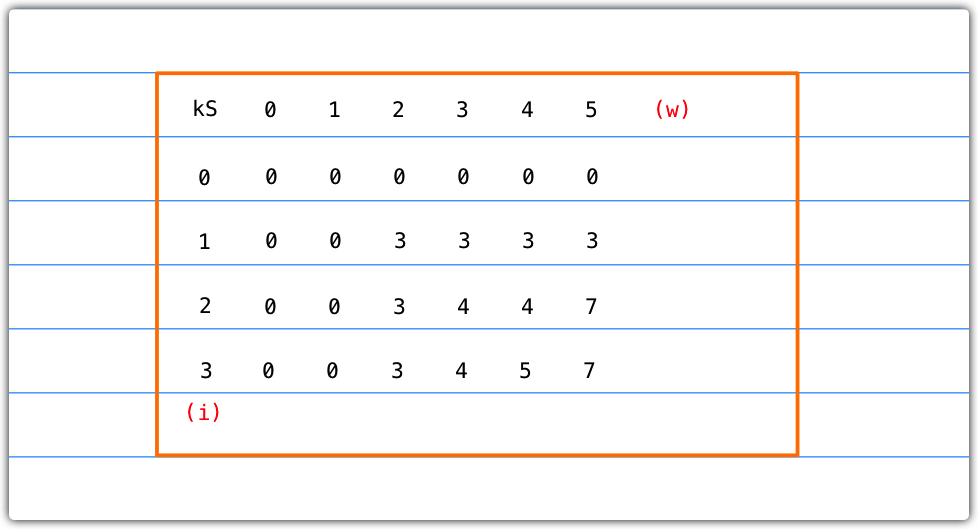• 物品数量和背包容量必须大于0，满足就执行while循环
• 当矩阵的[i][k]位置的元素不等于[i-1][k]位置的元素，就将其取出
• 取出后，改变i的值和w的值，即i--; k -= kS[i][k]

• 当i = 3, k = 5时，kS = 7, kS = 7两者相等，故向上查找，即i--
• 当i = 2, k = 5时，kS = 7, kS = 4两者不等，则构成组合，将其取出，即: [weights[i-1], values[i-1]] = [3, 4]，随后i--; k = k - kS[i][k] = 5 - kS = 5 - 3 = 2
• 此时，i = 1, k = 2, kS = 3, kS = 0两者不等，则构成组合，将其取出，即: [weights[i-1], values[i-1]] = [2, 3]，随后i--; k = k - kS[i][k] = 2 - 0 = 2，现在i = 0条件不满足，所有组合已找到退出循环。

##### 实现代码

/**
* 背包问题
* 即: 给定一个固定大小、能够携重量w的背包，以及一组有价值和重量的物品，找出一个最佳解决方案，使得装入背包的物品的总重量不超过w,且总价值最大
* @param capacity 背包容量
* @param weights 物品的重量
* @param values 物品的价值
* @param n 物品数量
*/
knapSack(capacity: number, weights: number[], values: number[], n: number): number {
// 声明并初始化需要寻找解决方案的矩阵
const kS: number[][] = [];
for (let i = 0; i <= n; i++) {
kS[i] = [];
}
for (let i = 0; i <= n; i++) {
for (let w = 0; w <= capacity; w++) {
if (i === 0 || w === 0) {
// 忽略矩阵的第一列和第一行，只处理索引不为0的列和行
kS[i][w] = 0;
} else if (weights[i - 1] <= w) {
// 物品i的重量必须小于约束
const a = values[i - 1] + kS[i - 1][w - weights[i - 1]];
const b = kS[i - 1][w];
console.log(i = ${i} :( a =${a} , b = ${b})); // 当找到可以构成解决方案的物品时，选择价值最大的那个 kS[i][w] = a > b ? a : b; } else { // 总重量超出背包能够携带的重量，忽略它用之前的值 kS[i][w] = kS[i - 1][w]; } } } DesignSkills.findValues(n, capacity, kS, weights, values); return kS[n][capacity]; } /** * 寻找背包物品可组成方案组合 * @param n 物品数量 * @param capacity 背包容量 * @param kS 背包最大价值矩阵 * @param weights 物品的重量 * @param values 物品的价值 * @private */ private static findValues(n: number, capacity: number, kS: number[][], weights: number[], values: number[]): void { let i = n; let k = capacity; console.log("构成解的物品"); // 物品数量和背包容量都大于0就执行循环 while (i > 0 && k > 0) { if (kS[i][k] !== kS[i - 1][k]) { // kS[i][k]位置的值不等于kS[i-1][k]位置的值就是一种方案,将其取出 console.log(物品${i} 可以是解的一部分 w,v: ${weights[i - 1]},${values[i - 1]};);
// 取出后i与k重新赋值
i--;
k -= kS[i][k];
} else {
// 向上找
i--;
}
}
}


##### 编写测试代码

import { DesignSkills } from "./lib/DesignSkills.ts";
const designSkills = new DesignSkills();
const values = [3, 4, 5],
weights = [2, 3, 4],
capacity = 5,
n = values.length;
console.log("所有组成方案的最大价值为", designSkills.knapSack(capacity, weights, values, n));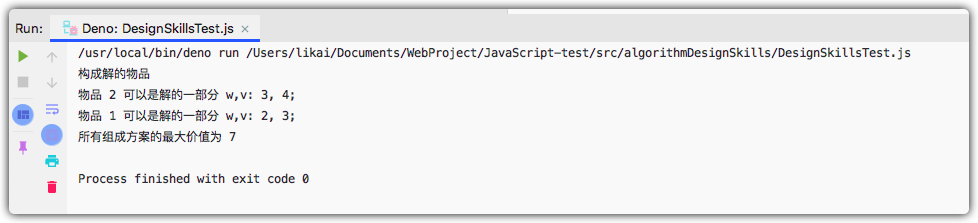#### 最长公共子序列

1. 需要两个参数：字符串1wordX、字符串2wordY
2. 声明两个辅助变量mn，用于接收两个字符串的长度。
3. 声明矩阵l，将其初始化为0
4. 遍历两个字符串，根据规则填充矩阵，填充规则如下：
(1). 当i==0 || j == 0l[i][j] = 0
(2). 当wordX[i - 1] == wordY[j - 1]l[i][j] = l[i - 1][j - 1] + 1
(3). 否则，声明两个辅助变量a,ba = [i - 1][j]; b = [i][j - 1]，取二者的最大值作为l[i][j]出的值，即l[i][j] = max(a, b)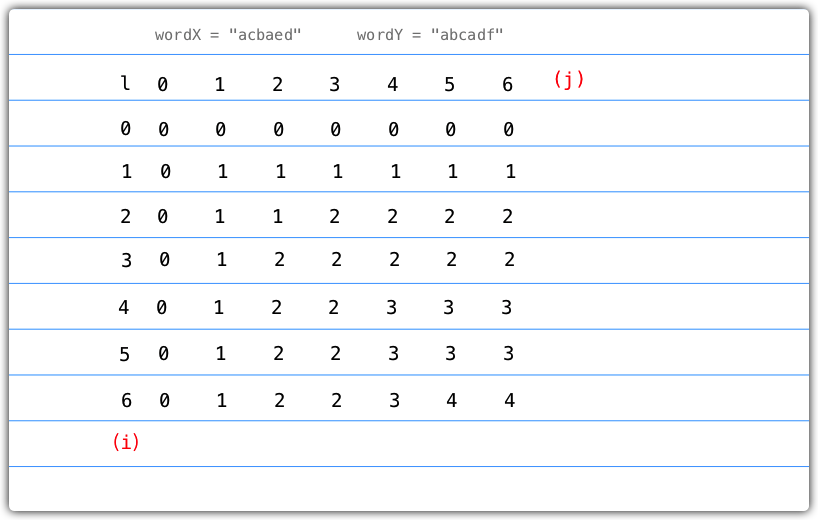1. i == 0 || j == 0S[i][j] = 0
2. wordX[i - 1] == wordY[j - 1]S[i][j] = "diagonal"
3. 否则，S[i][j] = l[i - 1][j]? "top" : "left"
| S | 0 | 1 | 2 | 3 | 4 | 5 | 6 | ( j ) |
| ----- | ---- | -------- | -------- | -------- | -------- | -------- | ---- | ----- |
| 0 | 0 | 0 | 0 | 0 | 0 | 0 | 0 | 0 |
| 1 | 0 | diagonal | left | left | diagonal | left | left | |
| 2 | 0 | top | top | diagonal | left | left | left | |
| 3 | 0 | top | diagonal | top | top | top | top | |
| 4 | 0 | diagonal | top | top | diagonal | left | left | |
| 5 | 0 | top | top | top | top | top | top | |
| 6 | 0 | top | top | top | top | diagonal | left | |
| ( i ) | | | | | | | | |

1. 如果solution[m][n] == "diagonal"，就将其取出并拼接，随后a--; b--
2. 如果solution[m][n] == "left"，则b--
3. 如果solution[m][n] == "top"，则a--

• m = 6时，其值为left，则b–
• 此时，m = 6,n = 5，其值为diagonal，则取出其值并拼接，即：answer = wordX[a-1] + answer = "d"。随后a--, b--
• 此时，m = 5, n=4，其值为top，则a--
• 此时，m = 4, n = 4，其值为diagonal，则取出，即：answer = wordX[a-1] + answer = "ad"，随后a--, b--
• 此时，m = 3, n = 3，其值为top，则a--
• 此时，m = 2, n = 3，其值为diagonal，则取出，即：answer = wordX[a-1] + answer = "cad"，随后a--, b--
• 此时m = 1, n = 2，其值为left，则b--
• 此时，m = 1, n = 1，其值为diagonal，则取出，即：answer = wordX[a-1] + answer = "acad"，随后a--, b--;
• 此时，m = 0, n = 0，组合推导完成，最长公共子序列: acad
##### 实现代码

/**
* 最长公共子序列
* @param wordX 字符串1
* @param wordY 字符串2
*/
lcs(wordX: string, wordY: string): number {
// 获取两个子序列的长度
const m = wordX.length;
const n = wordY.length;
// 声明并初始化二维数组，用于存放矩阵
const l: number[][] = [];
for (let i = 0; i <= m; i++) {
l[i] = [];
for (let j = 0; j <= n; j++) {
l[i][j] = 0;
}
}
for (let i = 0; i <= m; i++) {
for (let j = 0; j <= n; j++) {
if (i === 0 || j === 0) {
// i为0或者j为0，此处的格子就填充为0
l[i][j] = 0;
} else if (wordX[i - 1] === wordY[j - 1]) {
// 字符串1的i-1位置的值等于字符串2的j-1位置的值
l[i][j] = l[i - 1][j - 1] + 1;
} else {
// 否则，取出a、b位置的值，选出一个较大值进行填充
const a = l[i - 1][j];
const b = l[i][j - 1];
l[i][j] = a > b ? a : b;
}
}
}
// 最后一个格子即最长公共子序列的长度
return l[m][n];
}
/**
* 最长公共子序列解决方案
* @param wordX
* @param wordY
*/
lcsSolution(wordX: string, wordY: string): string {
// 获取两个子序列的长度
const m = wordX.length;
const n = wordY.length;
// 声明并初始化二维数组，用于存放矩阵
const l: number[][] = [];
// 存放用于推导组合的矩阵
const solution: string[][] = [];
for (let i = 0; i <= m; i++) {
l[i] = [];
solution[i] = [];
for (let j = 0; j <= n; j++) {
l[i][j] = 0;
solution[i][j] = "0";
}
}
for (let i = 0; i <= m; i++) {
for (let j = 0; j <= n; j++) {
if (i === 0 || j === 0) {
l[i][j] = 0;
} else if (wordX[i - 1] === wordY[j - 1]) {
l[i][j] = l[i - 1][j - 1] + 1;
// 如果相等则填充diagonal
solution[i][j] = "diagonal";
} else {
const a = l[i - 1][j];
const b = l[i][j - 1];
l[i][j] = a > b ? a : b;
// 如果相等就填充top否则填充left
solution[i][j] = l[i][j] == l[i - 1][j] ? "top" : "left";
}
}
}
// 求组合方案
return DesignSkills.getSolution(solution, wordX, m, n);
}
/**
* 根据最长公共子序列矩阵推导其组合方案
* @param solution 最长公共子序列矩阵
* @param wordX 字符串1
* @param m 矩阵的x轴指向
* @param n 矩阵的y轴指向
* @private
*/
private static getSolution(solution: string[][], wordX: string, m: number, n: number): string {
let a = m;
let b = n;
let x = solution[a][b];
while (x !== "0") {
if (solution[a][b] === "diagonal") {
// 相等就将其取出
a--;
b--;
} else if (solution[a][b] === "left") {
b--;
} else if (solution[a][b] === "top") {
a--;
}
// 重新赋值，继续下一轮循环
x = solution[a][b];
}
// 返回组合方案
}


##### 编写测试代码

import { DesignSkills } from "./lib/DesignSkills.ts";
const designSkills = new DesignSkills();
console.log(solution);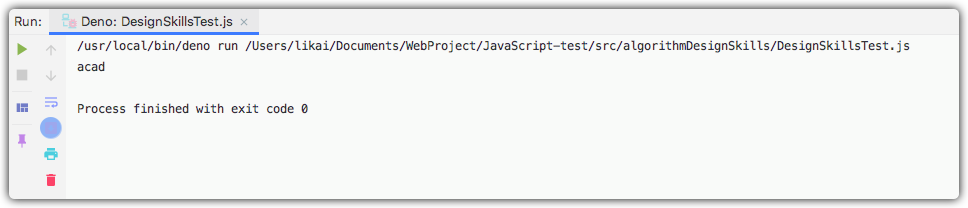#### 矩阵链相乘

##### 问题分析

// 描述了4个矩阵：
// A(10,100)
// B(100,5)
// C(5,50)
// D(50,1)
const p = [10, 100, 5, 50, 1]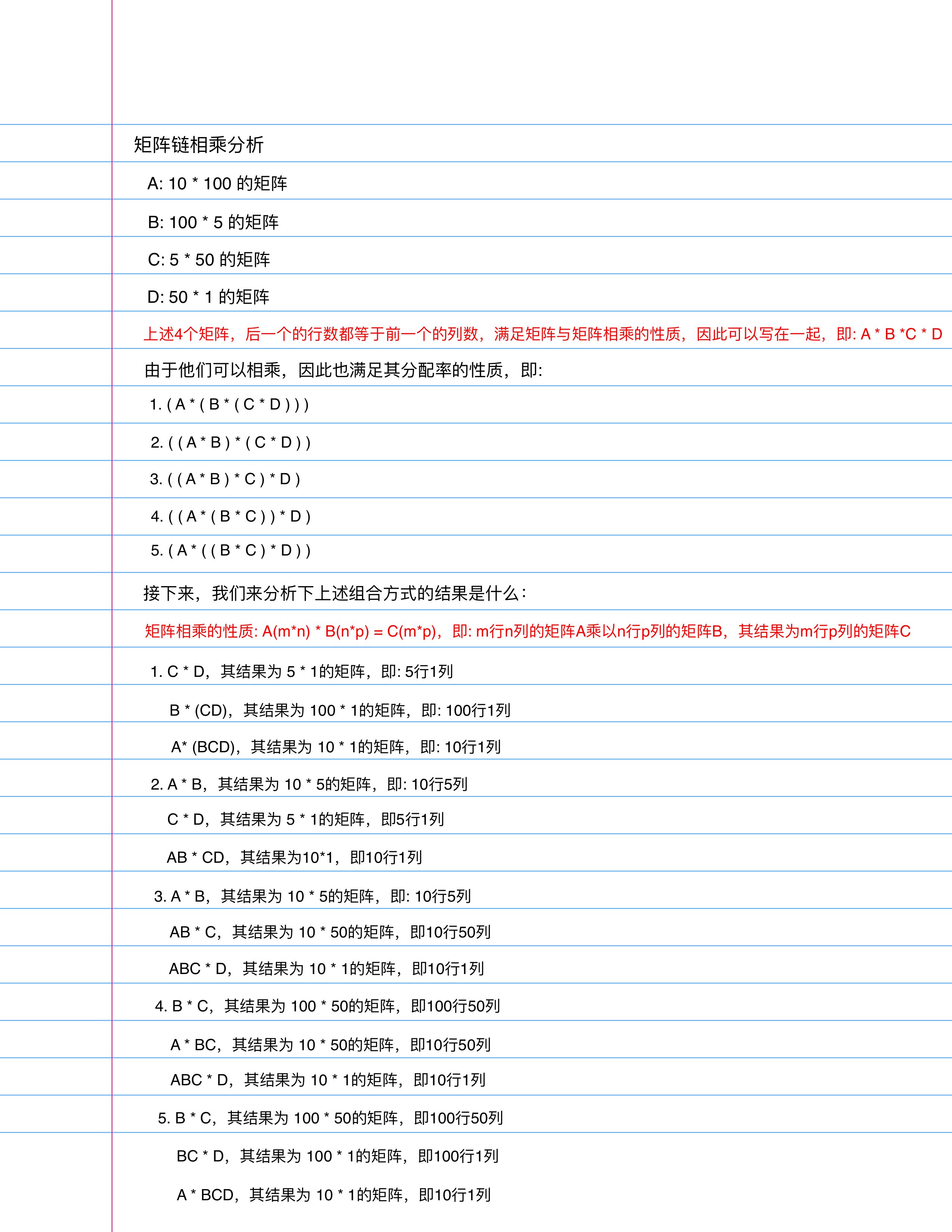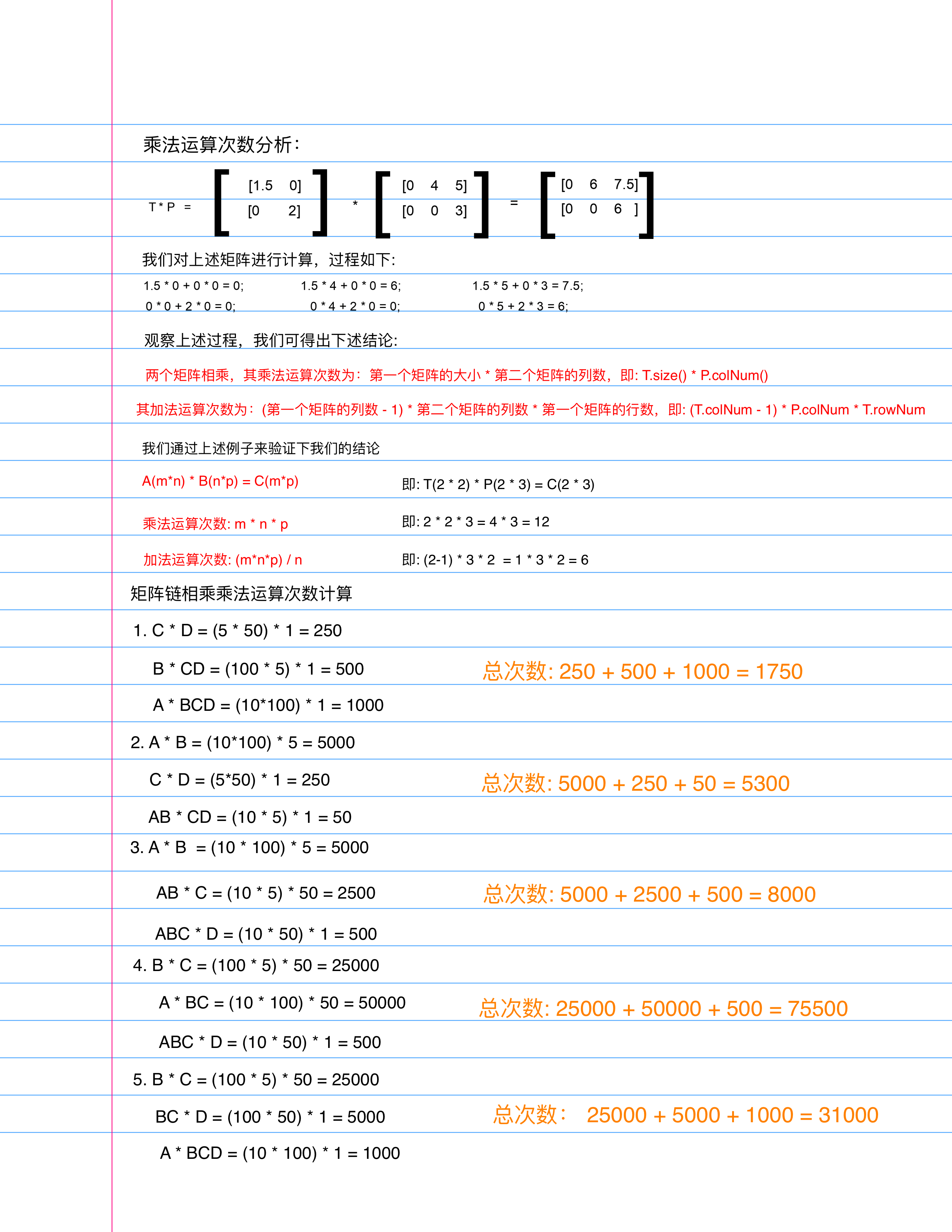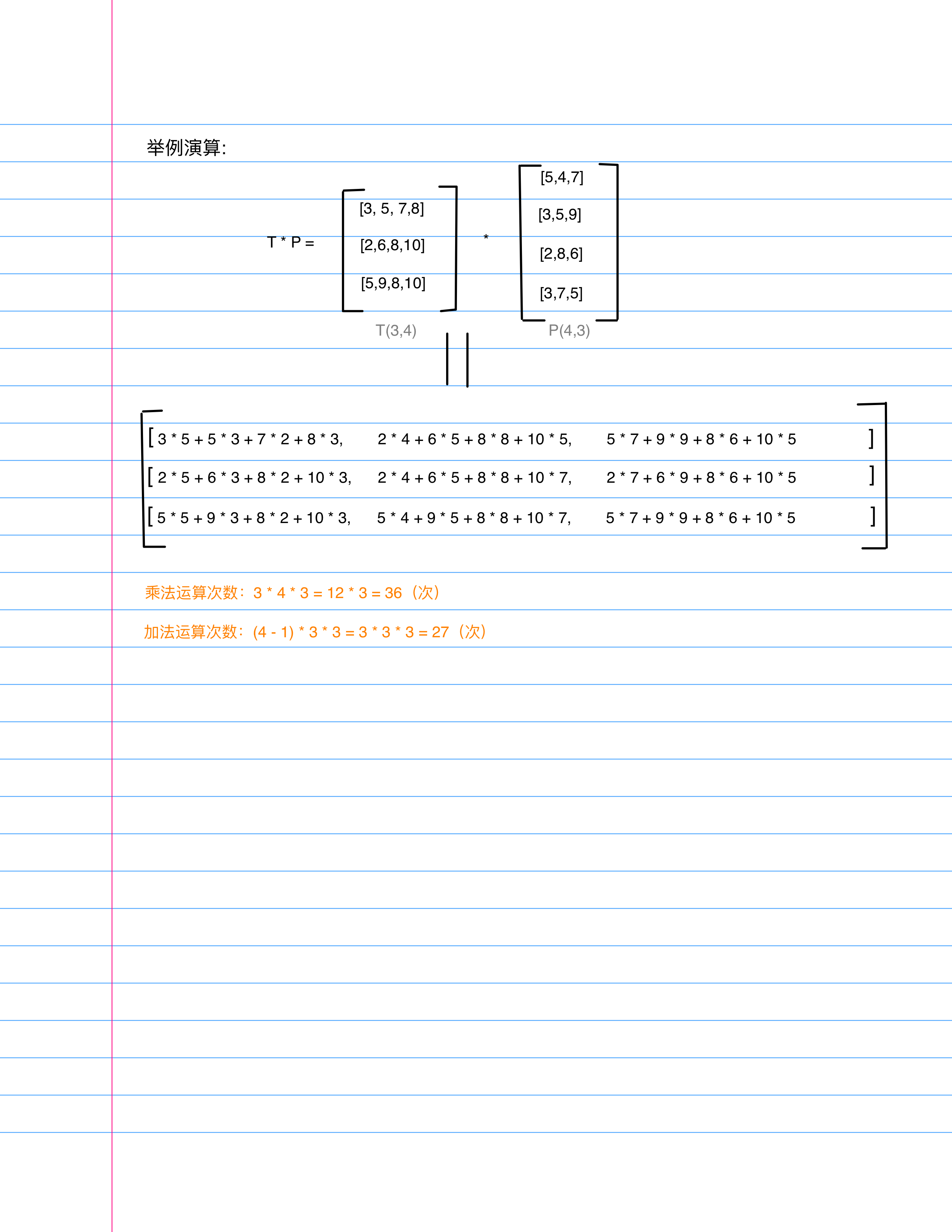##### 实现代码

// 矩阵链相乘
matrixChainOrder(p: number[]): number {
// 矩阵的长度
const n = p.length;
// 辅助矩阵：m存储最优次数
const m: number[][] = [];
const s: number[][] = [];
// 完整填充矩阵s
for (let i = 0; i <= n; i++) {
s[i] = [];
for (let j = 0; j <= n; j++) {
s[i][j] = 0;
}
}
// 对角线填充矩阵m
for (let i = 1; i <= n; i++) {
m[i] = [];
m[i][i] = 0;
}
// 更新矩阵m和s
for (let l = 2; l < n; l++) {
for (let i = 1; i <= n - l + 1; i++) {
// 计算要填充位置的值
const j = i + l - 1;
// 假设此处位置的值为最大
m[i][j] = Number.MAX_SAFE_INTEGER;
// console.table(m);
for (let k = 0; k <= j - 1; k++) {
// 计算公式：矩阵相乘得到的次数
const q = m[i][k] + m[k + 1][j] + p[i - 1] * p[k] * p[j];
// 如果计算出来的次数小于刚才假设的最大值就更新m矩阵和s矩阵的元素值
if (q < m[i][j]) {
// m[i][j]处的值就是计算出来的次数
m[i][j] = q;
s[i][j] = k;
}
}
}
}
// 打印矩阵相乘组合顺序
this.printOptimalParenthesis(s, 1, n - 1);
// m矩阵的第一行第i-1位置的值就是我们要的矩阵链相乘最少次数
return m[n - 1];
}
// 矩阵链相乘解决方案
printOptimalParenthesis(s: number[][], i: number, j: number): void {
if (i === j) {
console.log("A[" + i + "]");
} else {
console.log("(");
this.printOptimalParenthesis(s, i, s[i][j]);
this.printOptimalParenthesis(s, s[i][j] + 1, j);
console.log(")");
}
}

##### 编写测试代码

const p = [10, 100, 5, 50, 1];
console.log("矩阵链相乘其最优组合方式：");
const frequency = designSkills.matrixChainOrder(p);
console.log("矩阵链相乘其最少次数：", frequency);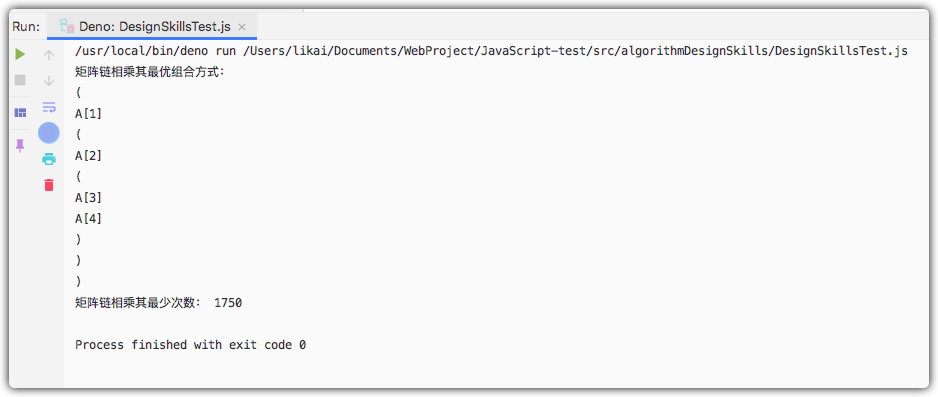## 代码地址

DesignSkills.ts & DesignSkillsTest.js

## 写在最后

• 文中如有错误，欢迎在评论区指正，如果这篇文章帮到了你，欢迎点赞和关注😊
• 本文首发于掘金，未经许可禁止转载💌
1